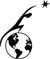# Appendix A-Moment of Inertia

����������� Tocompute the moment of inertia for a torus being revolved around the z axis, theequation I=(�R2+r2)M can be used, where R is the majorradius, r is the minor radius, and M is the total mass of the torus, or ρV[ref 47].In the below equations, R=major radius and R1=minor radius.Assuming the colony is a solid torus with density 100 kg/m3with a major radius of 2000 meters, and a minor radius of 255 (including theradiation shield), the moment of inertia can be found.� Thus the approximated moment of inertia is7.868x1017 kg·m2.

## Calculation

Volume of torus: V=2π2Rr2

I1=(�R2+r12)·M= [�·(2000m)2+(255m)2]·100kg/m3·2π2·2000m·(255m)2

 Curator: Al Globus If you find any errors on this page contact Al Globus.This site was hosted by the NASA Ames Research Center from 1994-2018 and is now hosted by: